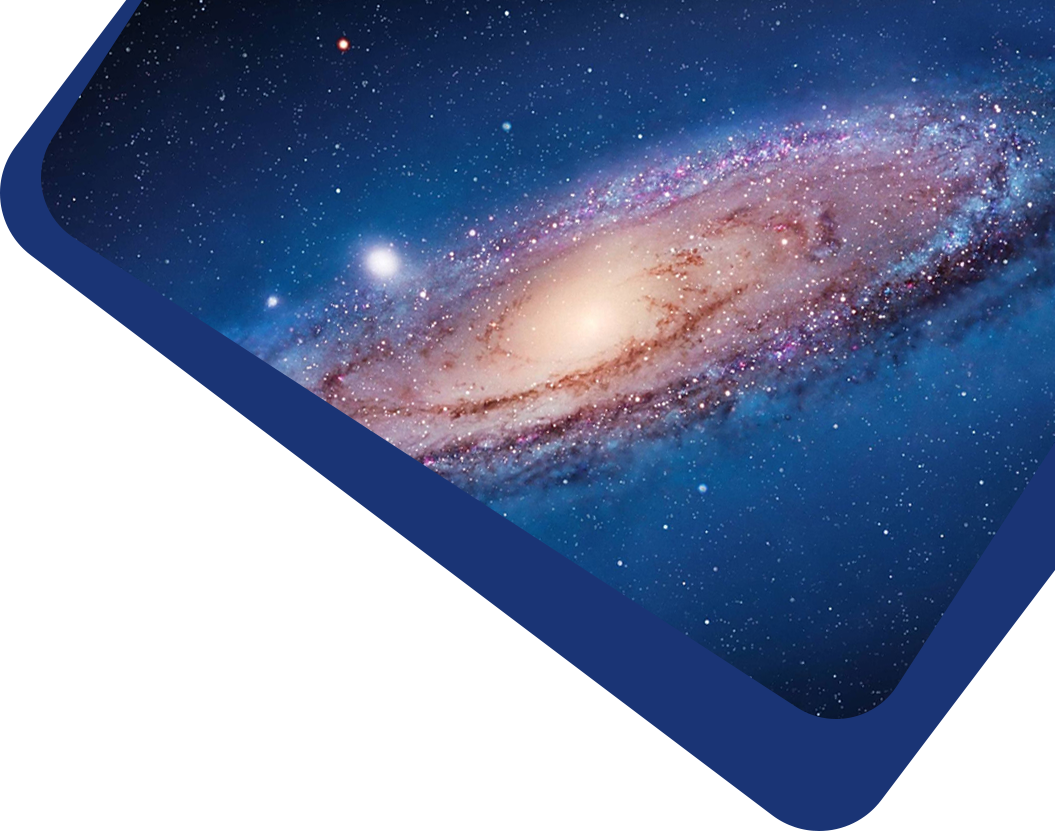Vol 22, No 6

## A Study on Low Frequency Electromagnetic Cyclotron Waves in the Solar Wind

#### Hai-Feng Yang, Guo-Qing Zhao, Heng-Qiang Feng, Gilbert Pi, Qiang Liu, Liang Xiang, Qiu-Huan Li

Abstract

Abstract According to Wind observations between 2005 and 2015, this paper investigates the dependences of the occurrence of low frequency electromagnetic cyclotron waves (ECWs) on the plasma parameters, the solar cycle, and the orientations of alpha-proton drift velocity ($$V_d$$) and the ambient magnetic field ($$B$$). The occurrence rates of ECWs with respect to six plasma parameters are calculated. Results show that the preferential conditions for generation of left-handed (LH) ECWs are higher proton temperature ($$T_p$$), higher proton velocity ($$V_p$$), lower proton density ($$N_p$$), stronger proton temperature anisotropy ($$T_{\perp}/T_{\parallel}$$), higher normalized alpha-proton drift velocity ($$V_d/V_A$$), and higher normalized alpha particle density ($$N_{\alpha}/N_p$$), where $$T_{\perp}$$ and $$T_{\parallel}$$ refer to proton temperatures perpendicular and parallel to B, and $$V_A$$ is the local Alfvén velocity. For right-handed (RH) ECWs, however, the dependences on these plasma parameters are not obvious. On the other hand, it is found that the occurrence rate of LH ECWs increases as the sunspot number decreases, and decreases as the sunspot number increases. Further investigation shows that the increased occurrence rate of LH ECWs is accompanied by an increase in the medians of $$V_p$$, $$V_d/V_A$$, and $$N_{\alpha}/N_p$$. For RH ECWs, the occurrence rate appears to be nearly unrelated to the sunspot number, suggesting a negligible correlation with the solar cycle. In addition, a modified angle is introduced to include the factors of orientation of B (anti-sunward or sunward) and angle between $$V_d$$ and B, simultaneously. It is found that the occurrence of LH ECWs has the strong preferential condition that $$V_d$$ is anti-sunward, while a different situation arises for RH ECWs. These results are discussed in the context of the temperature-anisotropy-driven instabilities with the effect of alpha particles.

Keywords

Keywords (Sun:) solar wind – instabilities – waves

Full Text
Refbacks

• There are currently no refbacks.